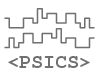PSICS - the Parallel Stochastic Ion Channel Simulator
previous ←   → next
Under development
Please let us know of errors, unclear text or if you have suggestions form improvements:Examples

PSICS comes with a range of built-in models. They can be run with the command-line argument "-e" followed by the name of the model to run. Some of the examples are also part of standard test cases that are run with specific options such as "-v" to run the rallpack validation examples.

For each model there is a single page summary that is generated automatically when the model is run.

• p18   Channel noise in dendrites
• cell-loc   Current clamp stimulation profile read from a file, varying the stimulation scale factor
• synapses   Simple cell with distributions of synapses and event generators to activate them
• minor   PSICS project generated from: CA1PyramidalCell.ncx
• params
• smalldt   PSICS project generated from: CA1PyramidalCell.ncx
• onsurface   Cable, 100um long, run for 250ms with different timesteps between 20 and 500 microseconds
• migliore-1a   Migliore et al CA1 pyramidal cell
• rallpack3   Cable with HH sodium and potassium channels, different timesteps
• cwvclamp   Chow and White (1996), Biophys J
• vcsteps
• rallpack1   Cable, 100um long, run for 250ms with different timesteps between 20 and 500 microseconds
• smartrec   Cell with smart recorders accessing channel population conductances independently
• ivconv
• ivconvm
• mean-variance-iv-stoch
• stochdet
• channel-functions   test for channels with xml coded functions
• stimtest   Current clamp stimulation profile read from a file, varying the stimulation scale factor
• functest   test for channels with xml coded functions
• migliore-pass   Migliore et al CA1 pyramidal cell
• psd
• cianmar30   Channel noise in dendrites
• mean-variance
• rallpack2   Regular branched cable with 9 levels of branching (512 terminals) varying timestep
• rallpack3-stoch   Cable with HH sodium and potassium channels
• rallpack3-stoch2   Cable with HH sodium and potassium channels, 20pS single channel conductance
• chowwhite   Chow and White (1996), Biophys J. Showing spontaneous spiking with varying numbers of channels
• multirec   Different ways of positioning recorders along the cell between defined points
• mainen
• soma-spikes-stochastic   Cable with channels only for first 50 um, varying time step, stochatic
• soma-spikes-continuous   Cable with channels only for first 50 um, varying time step
• p1-f1-mean-variance
• rallpack1-IE   Cable, 100um long, run for 250ms with different timesteps between 20 and 500 microseconds
• rallpack2-IE   Regular branched cable with 9 levels of branching (512 terminals) varying timestep
• singlecpt   Cable with channels only for first 50 um, varying time step
• synstim   Simple cell with distributions of synapses and event generators to activate them
• resonance   Spruston CA1 pyramidal neuron (with Ih)
• manychannels

Model specification samples

The files included below come from the rallpack3 example folder and illustrate how the various components of a model are specified. The full structure allowed for each type of model component is in the formats reference.

rallpack3/cell.xml

<CellMorphology id="cell">
<Point id="p0" x="0" y="0" z="0" r="0.5" />
<Point parent="p0" id="p1" x="1000" y="0" z="0" r="0.5" />
</CellMorphology>

rallpack3/environment.xml

<CellEnvironment id="environment">
<Ion id="LEAK" name="Non-specific leak" reversalPotential="-65mV" />
<Ion id="K" name="Potassium" reversalPotential="-77mV" />
<Ion id="Na" name="Sodium" reversalPotential="50mV" />
</CellEnvironment>

rallpack3/membrane.xml

<CellProperties id="membrane" cytoplasmResistivity="100ohm_cm" membraneCapacitance="1uF_per_cm2">

<ChannelPopulation channel="leak" density="25per_um2" />

<ChannelPopulation channel="HH_Na" density="60per_um2" />
<ChannelPopulation channel="HH_K" density="18per_um2" />

</CellProperties>

rallpack3/HH_K.xml

<KSChannel id="HH_K" permeantIon="K" gSingle="20pS">

<KSComplex id="n" instances="4">
<ClosedState id="c" />
<OpenState id="o" />
<ExpLinearTransition from="c" to="o" rate="0.1per_ms" midpoint="-55.mV" scale="10mV" />
<ExpTransition from="o" to="c" rate="0.125per_ms" midpoint="-65.mV" scale="-80mV" />
</KSComplex>

</KSChannel>

rallpack3/HH_Na.xml

<KSChannel id="HH_Na" permeantIon="Na" gSingle="20pS">

<KSComplex id="m" instances="3">
<ClosedState id="c" />
<OpenState id="o" />
<ExpLinearTransition from="c" to="o" rate="1.per_ms" midpoint="-40.mV" scale="10mV" />
<ExpTransition from="o" to="c" rate="4.per_ms" midpoint="-65.mV" scale="-18mV" />
</KSComplex>

<KSComplex id="h">
<ClosedState id="c" />
<OpenState id="o" />
<ExpTransition from="c" to="o" rate="0.07per_ms" midpoint="-65.mV" scale="-20.mV" />
<SigmoidTransition from="o" to="c" rate="1per_ms" midpoint="-35mV" scale="10mV" />
</KSComplex>

</KSChannel>

rallpack3/recording.xml

<Access id="recording" recordClamps="false">

<CurrentClamp label="start" at="p0" lineColor="red" hold="0.1nA" record="true">

</CurrentClamp>

<VoltageRecorder label="end" at="p1" lineColor="blue" />

</Access>

rallpack3/run.xml

<PSICSRun timeStep="0.1ms" runTime="250ms" startPotential="-65mV" morphology="cell" environment="environment" properties="membrane" access="recording" stochThreshold="0">
<StructureDiscretization baseElementSize="1um" />

<info>Cable with HH sodium and potassium channels, different timesteps</info>

<RunSet vary="timeStep" values="[50, 100]us" filepattern="ts-\$" />

<ViewConfig>
<LineGraph width="500" height="400">
<XAxis label="time / ms" />
<YAxis label="potential / mV" />

<Line file="ref_axon_0_neuron.txt" color="white" width="1" rescale="[1000, 1000.]" />
<Line file="ref_axon_x_neuron.txt" color="white" width="1" rescale="[1000, 1000.]" />

<LineSet file="ts-10.txt" color="red" />
<LineSet file="ts-20.txt" color="blue" />
<LineSet file="ts-50.txt" color="cyan" />
<LineSet file="ts-100.txt" color="green" />

<View id="whole" />
<View id="start" xmin="0." xmax="30." ymin="-100." ymax="80." />
<View id="end" xmin="210." xmax="255." ymin="-100." ymax="80." />

</LineGraph>
</ViewConfig>

</PSICSRun>
previous ←   → next